##Rectangular Hyperbola

The simplest one is the hyperbola defined through the graph of the function y = 1/x (blue). The rectangular hyperbola in general is defined as an hyperbola which has orthogonal asymptotic lines. All rectangular hyperbolas are congruent to the hyperbolas resulting from the previous one through a homothety centered at O (gray). The focal point F1 of the hyperbola results by multiplying OA with sqrt(2). The other focal point F2 is symmetric to F1 w.r. to O.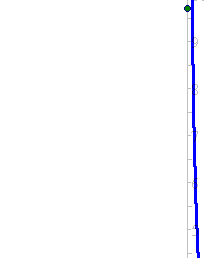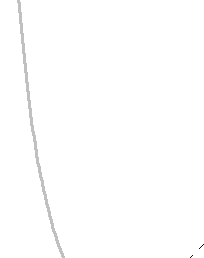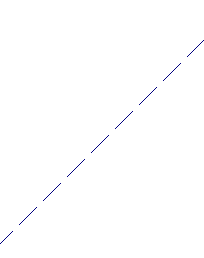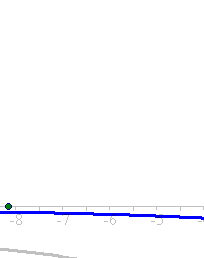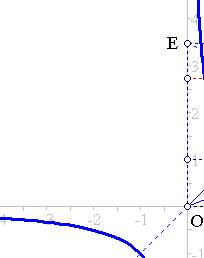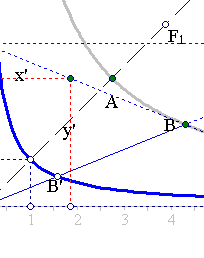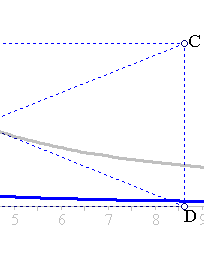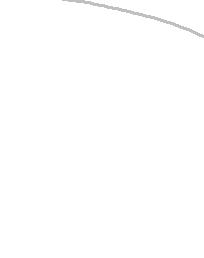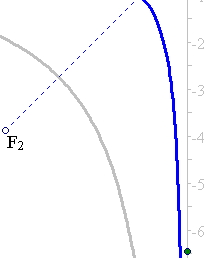Define the symmetric C of O w.r. to B(x0,y0) and build the rectangle ODCE. The diagonal DE is tangent to the hyperbola at B. In fact, at B it has a common point with the hyperbola. Then, an arbitrary point (x',y') on the line DE has y'/(2x0-x') = y0/x0 = a2/x02, where x0*y0 = a2 is the equation of the hyperbola. Thus y' = (a2(2x0-x'))/x02 < a2/x' <==> 0 < (x0-x')2 , which means that y' is below the hyperbola point (x', a2/x'). AD having only one common point (B) with the hyperbola is tangent to it. It follows:
1) The tangent at B defines a segment DE between the asymptotic lines which is bisected by B.
2) The area of all [asymptotic] triangles ODE (depend on B) is constant and equal to 2x0y0 = 2a2 = OA2.
3) Since every hyperbola can be obtained from the standard one (defined through y=1/x) through an affinity, the two previous results are valid for all hyperbolas (see HyperbolaFromRectangular.html ).
The rectangular hyperbola is among the hyperbolas the analogon of the circle among ellipses. There are though significant differences. For example, three points in general position define a unique circle containing them, whereas rectangular hyperbolas are uniquely determined by four points in general position (and non-orthocentric).

AsymptoticTriangle.html
Hyperbola.html
HyperbolaAsymptoticProperty.html
HyperbolaAsymptotics.html
HyperbolaFromRectangular.html
OrthoRectangular.html
RectangularAsProp.html
RectHyperbola.html
RectHyperbolasTangent4Lines.html
RectHypeImpossible.html
RectHypeRelation.html
RectHyperbolaTriaInscribed.html
RectHypeThroughFourPts.html